## Friday, April 30, 2010

### 周五--- I ♥ Cheezels

^-^

^口^~~~

"吃饱饭还吃cheezels??"

------- ------- -------

=0=

v
v
v

v
v
v

on9或看戏到一半

=___=

v
v
v

(可是却有很多快熟面==)

^^

=-=

(什么事都要说一餐)

## Thursday, April 29, 2010

### 电脑回来了^^

v
v
v
Lumix G2

=-=

ipoh有一间"印度戏院"

------ ------ ------

^-^

## Wednesday, April 28, 2010

### 小说更新^^

《把爱牵起♥》- 第十章

------- ------- -------

==''

anyway~

^^

^-^

"Wow~原来我是那么有天分的"

^++^

## Monday, April 26, 2010

### 我们的情绪表达方式♥

"亲爱的,你在干嘛呢^^?"
"老公在弄着电脑的东西^^老婆呢？"

-----------------------

"老公今晚想谈电话吗^^?"
"不了~今天有累了~"

-----------------------

"你不要这样好吗?!"
"我怎么样了?!?!是你在无理取闹！"
"你根本不明"
"我不想跟你谈了"

-----------------------### 我是这样的女生

^^## Friday, April 23, 2010

### 我是Avatar纳美族 ? (圆梦)

《把爱牵起♥》- 第九章

^^

------- ------- ------- -------

"对地球仁慈,也就是对自己仁慈"

v
v
v
v
v
v

(有人说像猫=^=''''')v
v
v
v
vps的过程还挺麻烦的

^~^## Thursday, April 22, 2010

### 我拿到^^/ 《爱情,来了》

^-^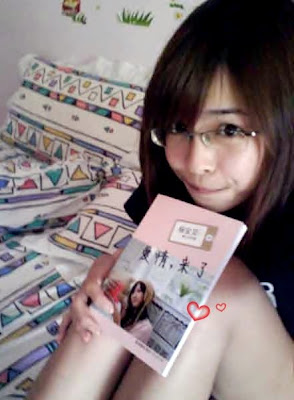v
v
v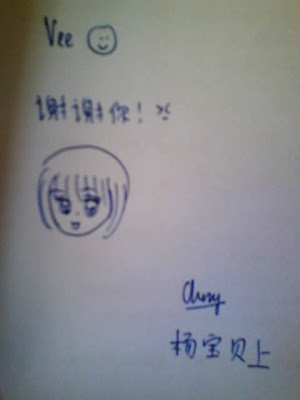^^

^-^

(我feel到的)

(晓珊&梁汉良在学海有写专栏)

(这是我从来就没有试过的)

《爱情,来了》## Wednesday, April 21, 2010

### 不冷的 云顶之旅^-^

(胃痛哪来的心情==)

^^

=-=

(在ipoh rm4的炒饭已经会被人骂说贵)

(不冷也就也不爽了)

v
v
vv
v
v
vv
v
vv
v
v
vv
v
v

(路人：这样都好拍？)hoho
v
v
v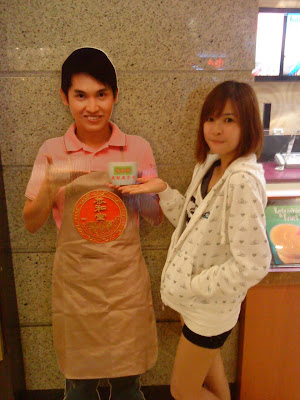^+++++^
(依然很多人在看==)^^

v
v
v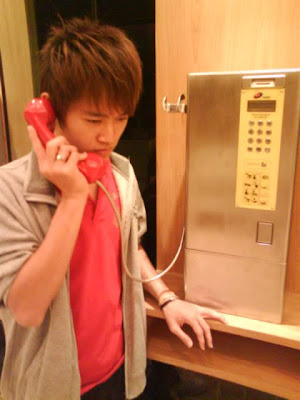ofcourse少不了合照^^
v
v
v
hehe^++^v
v
vi love it~!
v
v
vv
v
vv
v
v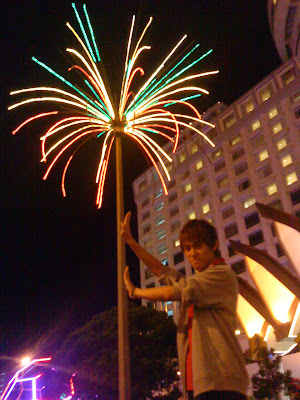^^

v
vv
v
v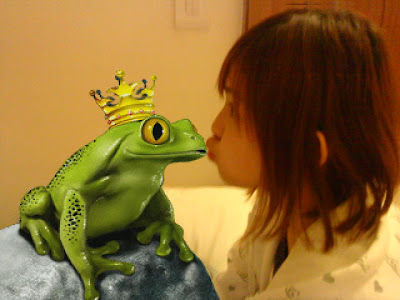v
v
vv
v
v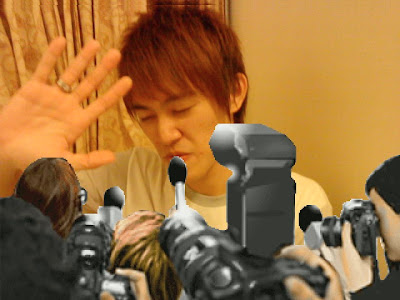v
v
v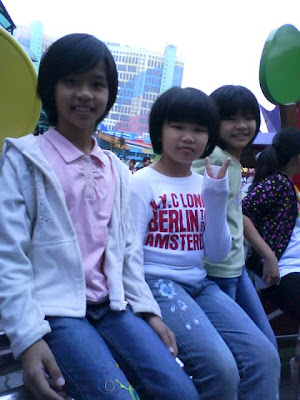v
v
v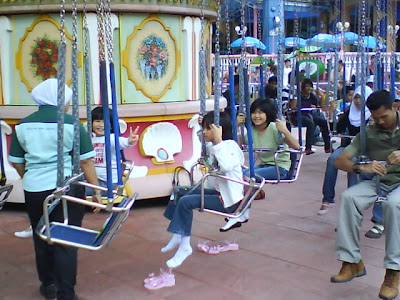v
v
vv
v
v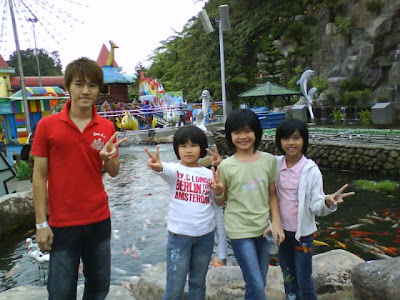^^
v
v
v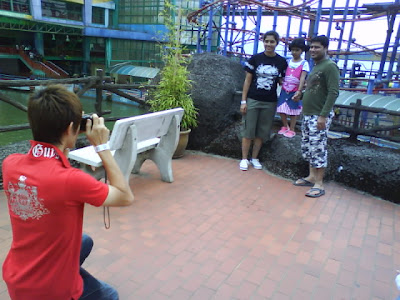v
v
v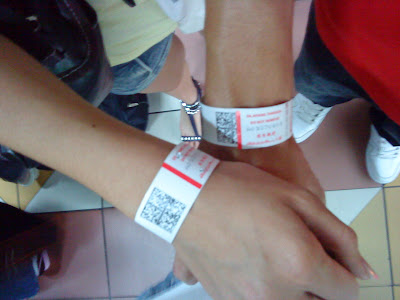v
v
v
v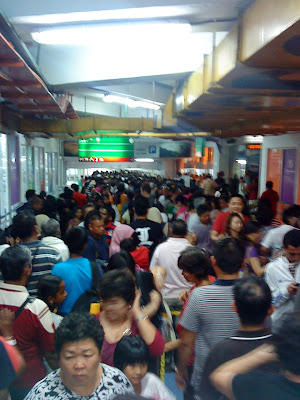v
v
v^^

v
vBYE BYE云顶^^/
v
v
vhoho

^-^